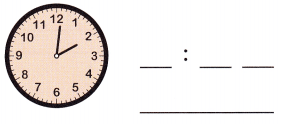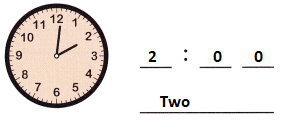# McGraw Hill Math Grade 3 Chapter 9 Lesson 1 Answer Key Tell and Write Time

## McGraw-Hill Math Grade 3 Answer Key Chapter 9 Lesson 1 Tell and Write Time

Write

Look at the clock. Write the time using numbers and words.

Question 1.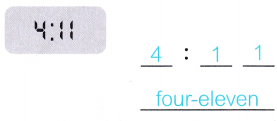Question 2.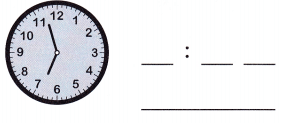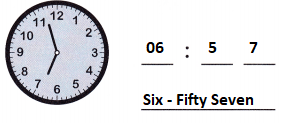Explanation:
By seeing the above clock we can see that the hour hand is about 7 and the minute hand is two points after 11.
So, the time is 6:57

Question 3.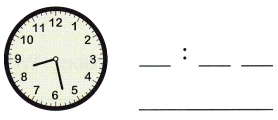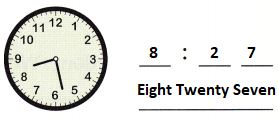Explanation:
By seeing the above clock we can see that the hour hand is on 8 and the minute hand is about 6.
So, the time is 8:27

Question 4.